# Chemical Kinetics - JEE Main Previous Year Questions with Solutions

JEE Main Previous Year Papers Questions of Chemistry with Solutions are available at eSaral. Practicing JEE Mains chapter wise questions of Chemistry will help the JEE aspirants in realizing the question pattern as well as help in analyzing weak & strong areas.   Simulator   Previous Years AIEEE/JEE Mains Questions
Q. The half life period of a first order chemical reaction is 6.93 minutes. The time required for the completion of 99% of the chemical reaction will be (log 2 = 0.301) :- (1) 46.06 minutes             (2) 460.6 minutes            (3) 230.3 minutes              (4) 23.03 minutes [aieee-2009]
Ans. (1)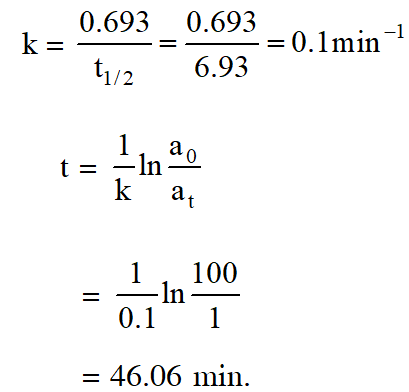Q. The time for half life period of a certain reaction A Products is 1 hour, when the initial concentration of the reactant 'A' is 2.0 mol L–1, How much time does it take for its concentration to come from 0.50 to 0.25 mol L–1 if it is a zero order reaction? (1) 1 h (2) 4 h (3) 0.5 h (4) 0.29. [aieee-2010]
Ans. (4) For zero order reaction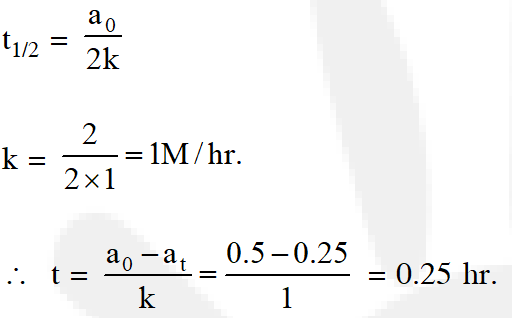Q. Consider the reaction: $\mathrm{Cl}_{2}(\mathrm{aq})+\mathrm{H}_{2} \mathrm{S}(\mathrm{aq}) \rightarrow \mathrm{S}(\mathrm{s})+2 \mathrm{H}^{+}(\mathrm{aq})+2 \mathrm{Cl}^{-}(\mathrm{aq})$ The rate equation for this reaction is rate = $\mathrm{k}\left[\mathrm{Cl}_{2}\right]\left[\mathrm{H}_{2} \mathrm{S}\right]$ Which of these mechanisms is/are consistent with this rate equation?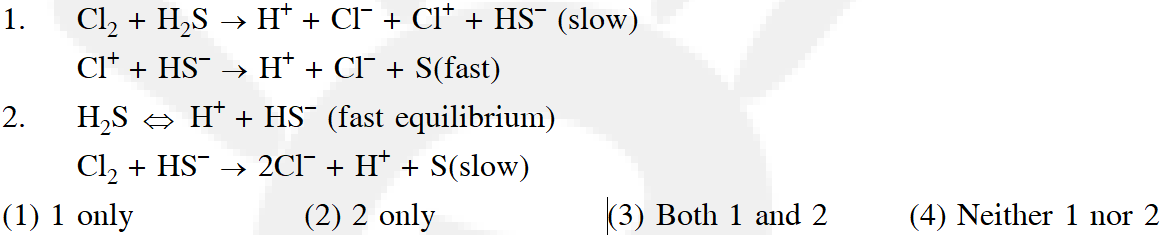[aieee-2010]
Ans. (1)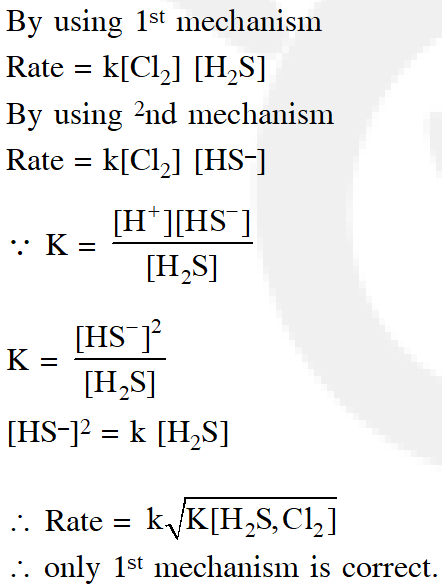Q. A reactant (A) forms two products :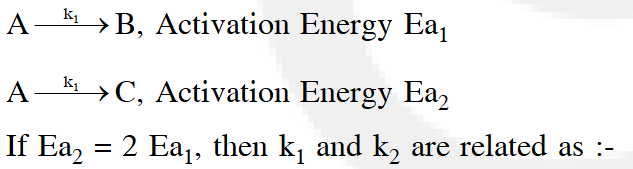(1) $\mathrm{k}_{1}=2 \mathrm{k}_{2} \mathrm{e}^{\mathrm{E}_{2} / \mathrm{RT}}$ (2) $\mathrm{k}_{1}=\mathrm{k}_{2} \mathrm{e}^{\mathrm{Ea}_{1} / \mathrm{RT}}$ (3) $\mathrm{k}_{2}=\mathrm{k}_{1} \mathrm{e}^{\mathrm{Ea}_{2} / \mathrm{RT}}$ (4) $\mathrm{k}_{1}=\mathrm{A} \mathrm{k}_{2} \mathrm{e}^{\mathrm{Ea}_{1} / \mathrm{RT}}$ [aieee-2011]
Ans. (2)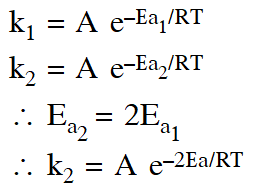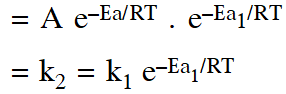Q. The rate of a chemical reaction doubles for every $10^{\circ} \mathrm{C}$ rise of temperature. If the temperature is raised by $50^{\circ} \mathrm{C}, \mathrm$ the rate of the reaction increases by about :- (1) 32 times            (2) 64 times             (3) 10 times             (4) 24 times [aieee-2011]
Ans. (1) $\mathrm{k}_{2}=\mathrm{k}_{1}(2)^{5}=32 \mathrm{k}_{1}$
Q. For a first order reaction, (A)  products, the concentration of A changes from 0.1M to 0.025M in 40 minutes. The rate of reaction when the concentration of A is 0.01 M is : (1) $1.73 \times 10^{-4} \mathrm{M} / \mathrm{min}$ (2) $1.73 \times 10^{-5} \mathrm{M} / \mathrm{min}$ (3) $3.47 \times 10^{-4} \mathrm{M} / \mathrm{min}$ (4) $3.47 \times 10^{-5} \mathrm{M} / \mathrm{m}$ [aieee-2012]
Ans. (3)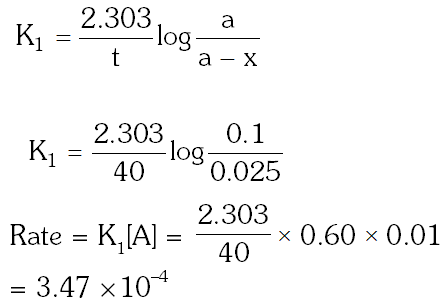Q. The rate of a reaction doubles when its temperature changes from 300 K to 310 K. Activation energy of such a reaction will be $\left(\mathrm{R}=8.314 \mathrm{JK}^{-1} \mathrm{mol}^{-1} \text { and } \log 2=0.301\right)$ (1) $53.6 \mathrm{kJ} \mathrm{mol}^{-1}$ (2) $48.6 \mathrm{kJ} \mathrm{mol}^{-1}$ (3) $58.5 \mathrm{kJ} \mathrm{mol}^{-1}$ (4) $60.5 \mathrm{kJ} \mathrm{mol}^{-1}$ [J-main 2013]
Ans. (1)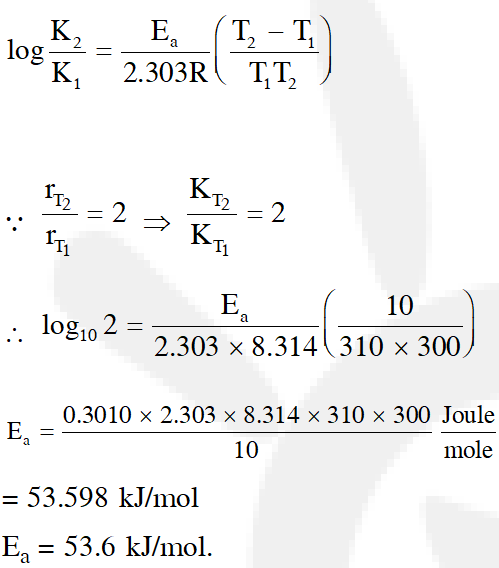Q. For the non-stoichiometre reaction 2A + B C + D, the following kinetic data were obtained in three separate experiments, all at 298 K.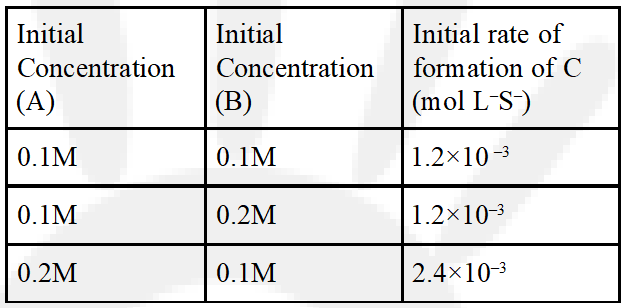(1) $\frac{\mathrm{dc}}{\mathrm{dt}}=\mathrm{k}[\mathrm{A}][\mathrm{B}]^{2}$ (2) $\frac{\mathrm{dc}}{\mathrm{dt}}=\mathrm{k}[\mathrm{A}]$ (3) $\frac{\mathrm{dc}}{\mathrm{dt}}=\mathrm{k}[\mathrm{A}][\mathrm{B}]$ (4) $\frac{\mathrm{dc}}{\mathrm{dt}}=\mathrm{k}[\mathrm{A}]^{2}[\mathrm{B}]$ [J-main 2014]
Ans. (2)
Q. Higher order (>3) reactions are rare due to :- (1) shifting of equilibrium towards reactants due to elastic collision (2) loss of active species on collision (3) low probability of simultaneous collision of all the reacting species (4) increase in entropy and activation energy as more molecules are involved. [JEE-MAIN-(Offline) 2015]
Ans. (3) Higher order (>3) reaction are rare due to low probability of simulatneous collision of more than three molecuels.
Q. The reaction $2 \mathrm{N}_{2} \mathrm{O}_{5}(\mathrm{g}) \rightarrow 4 \mathrm{NO}_{2}(\mathrm{g})+\mathrm{O}_{2}(\mathrm{g})$ follows first order kinetics. The pressure of a vessel containing only $\mathrm{N}_{2} \mathrm{O}_{5}$ was found to increase from 50 mm Hg to 87.5 mm Hg in 30 min. The pressure exerted by the gases after 60 min. will be (Assume temperature remains constant) (1) 106.25 nm Hg (2) 116.25 nm Hg (3) 125 mm Hg (4) 150 mm Hg [JEE-MAIN (Online)2015]
Ans. (2)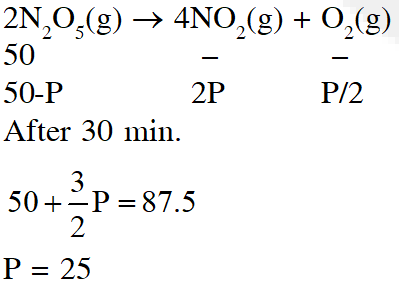Q. For the equilibrium, $\mathrm{A}(\mathrm{g}) \square \mathrm{B}(\mathrm{g}), \Delta \mathrm{H}$ is is –40 kJ/mol. If the ratio of the activation energies of the forward $\left(\mathrm{E}_{\mathrm{f}}\right)$ and reverse $\left(\mathrm{E}_{\mathrm{b}}\right)$ reactions is $\frac{2}{3}$ then :- (1) $\mathrm{E}_{\mathrm{f}}=60 \mathrm{kJ} / \mathrm{mol} ; \mathrm{E}_{\mathrm{b}}=100 \mathrm{kJ} / \mathrm{mol}$ $|(2) \mathrm{E}_{\mathrm{f}}=30 \mathrm{kJ} / \mathrm{mol} ; \mathrm{E}_{\mathrm{b}}=70 \mathrm{kJ} / \mathrm{mol}$ (3) $\mathrm{E}_{\mathrm{f}}=80 \mathrm{kJ} / \mathrm{mol} ; \mathrm{E}_{\mathrm{b}}=120 \mathrm{kJ} / \mathrm{mol}$ (4) $\mathrm{E}_{\mathrm{f}}=70 \mathrm{kJ} / \mathrm{mol} ; \mathrm{E}_{\mathrm{b}}=30 \mathrm{kJ} / \mathrm{mol}$ [JEE-MAIN (Online)2015]
Ans. (3)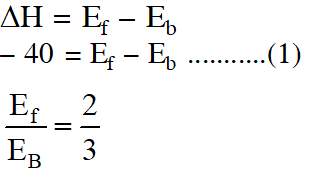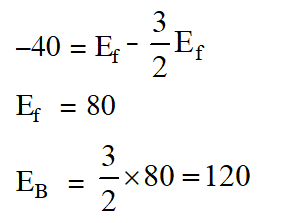Q. Decomposition of $\mathrm{H}_{2} \mathrm{O}_{2}$ follows a first order reaction. In fifty minutes the concentration of $\mathrm{H}_{2} \mathrm{O}_{2}$ decreases from 0.5 to 0.125 M in one such decomposition. When the concentration of $\mathrm{H}_{2} \mathrm{O}_{2}$ reaches 0.05 M, the rate of formation of $\mathrm{O}_{2}$ will be :-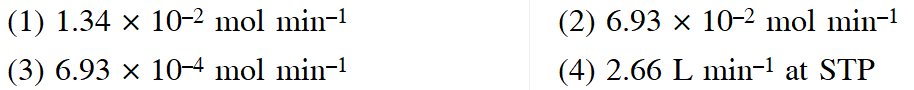[JEE - Main 2016]
Ans. (3) $\mathrm{H}_{2} \mathrm{O}_{2(\mathrm{aq})} \longrightarrow \mathrm{H}_{2} \mathrm{O}_{(\mathrm{aq})}+\frac{1}{2} \mathrm{O}_{2}(\mathrm{g})$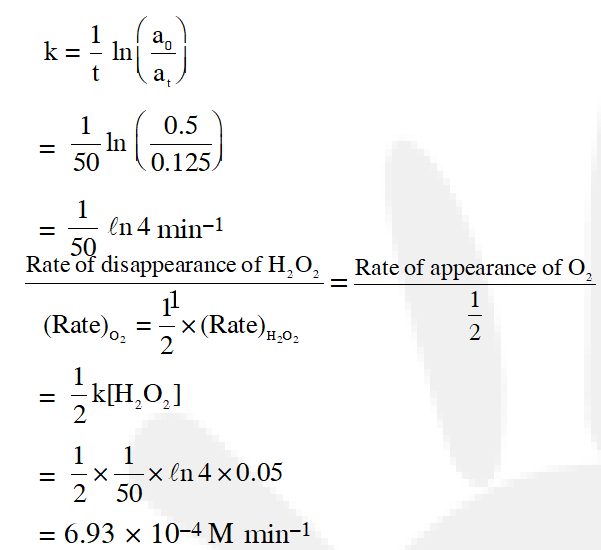Q. Two reactions R1 and R2 have identical pre-exponential factors. Activation energy of $\mathrm{R}_{1}$ exceeds that of $\mathrm{R}_{2}$ by 10 kJ $\mathrm{mol}^{-1}$. If k1 and k2 are rate constants for reactions $\mathrm{R}_{1}$ and $\mathrm{R}_{2}$ respectively at 300 K, then ln $\left(\mathrm{k}_{2} / \mathrm{k}_{1}\right)$ is equal to :- (1) 8 (2) 12 (3) 6 (4) 4 [JEE - Main 2017]
Ans. (4) From arrhenius equation,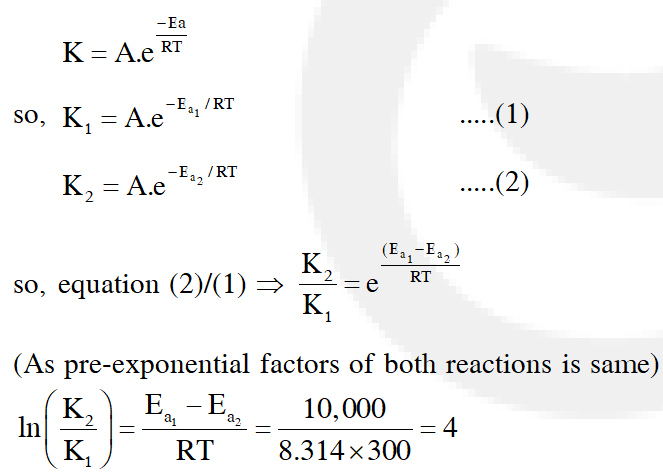Q. At $518^{\circ} \mathrm{C}$, the rate of decomposition of a sample of gaseous acetaldehyde, initially at a pressure of 363 Torr, was 1.00 Torr $\mathrm{s}^{-1}$ when 5% had reacted and 0.5 Torr s–1 when 33% had reacted. The order of the reaction is : (1)3 (2) 1 (3) 0 (4) 2 [JEE - Main 2018]
Ans. (4)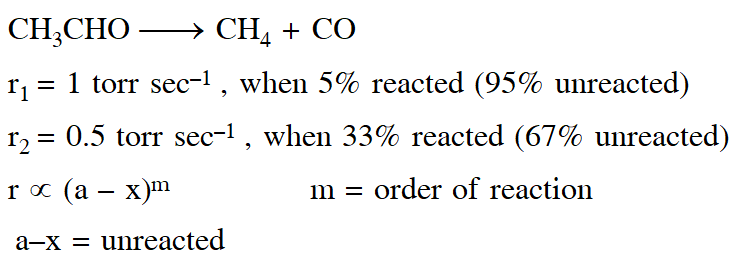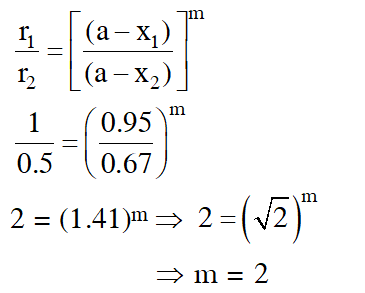Sarang C 94%
Dec. 27, 2021, 2:38 p.m.
gavrav sir aaj check mat karo na sir plzzzzzzzzzzzz
a
July 24, 2021, 10:19 p.m.
We want 2019 and 2020 bits also beaut
a
July 24, 2021, 10:18 p.m.
We want 2019 and 2020 bits also .
vijay
June 13, 2021, 5:33 a.m.
latest questions plz
June 8, 2021, 1:19 p.m.
Seriously some question are not interesting
Kamal AB
May 4, 2021, 10:49 a.m.
In 5th question what is that. Yellow marked lineee can anybody reply
naam janke kya kroge
Feb. 15, 2021, 5:36 p.m.
Maja nahi aa raha
Feb. 15, 2021, 5:11 p.m.
Thank you for giving this special opportunity to solve this paper, becoz you opened my eyes. Th
Rjsv
Feb. 10, 2021, 8:24 a.m.
Pls add 2019 and 2020 wuestions
Chandu mudhiraj
Jan. 27, 2021, 6:23 a.m.
Nyc questions but we need more questions
Oct. 23, 2020, 3:49 p.m.
hi this is good
Vineet
Sept. 28, 2020, 7:21 a.m.
Now I am appearing for Jee mains 2021 January and I like to use this .
Devanshu sharma
Sept. 16, 2020, 7:49 p.m.
very nice but we need more question
Nirmal
Sept. 14, 2020, 7:35 p.m.
10 hours done
Lahari
Sept. 1, 2020, 4:28 p.m.
tnx... very helpful for last min revision
Thangellapally Sai Teja
Aug. 31, 2020, 10:52 a.m.
Good questions !!😃
srk
Aug. 30, 2020, 9:03 a.m.
chAK DE india
Akhil
Aug. 29, 2020, 8:13 a.m.
Nice
pranith
Aug. 28, 2020, 3:33 p.m.
very helpful and make it better
Vaishnaav
Aug. 24, 2020, 6:15 a.m.
Is 2015 ...que ans was right
Aug. 23, 2020, 3:42 p.m.
Very useful for jee exam
Syed Ruvii
Aug. 21, 2020, 3:37 p.m.
Answer to the jee main 2015 question is given wrong.....right one is 106.25
Srigowthami
Aug. 20, 2020, 8:32 p.m.
Thank u so much..helped me a lot ....
Harshitha
Aug. 19, 2020, 8:25 p.m.
It's very much useful It helps to know our weak areas and improve better
Divyansh
Aug. 18, 2020, 12:09 p.m.
Thanku so mch for this
Aug. 14, 2020, 10:25 p.m.
helphul
Rocky
Aug. 13, 2020, 11:59 a.m.
Excellent questions👍👍
Karthik
Aug. 10, 2020, 11:27 a.m.
👍 questions interesting
Ansari zaid
July 27, 2020, 12:33 a.m.
Nice questions
P K
July 15, 2020, 5:39 p.m.
Thanks again for your Lovely wishes to you and
Mothilal
July 12, 2020, 10:30 a.m.
Its nice helped for mock test
S
July 11, 2020, 11:27 p.m.
Thanks
sahil
July 11, 2020, 7:40 a.m.
your log value in 1st ques is in corrrect
Nanda
July 5, 2020, 7:52 p.m.
God ones
July 4, 2020, 5:30 p.m.
we have begun the hunt for talented individuals . hello ! CICADA 101 we are the one
Yas
July 1, 2020, 9:15 p.m.
Good and maintain the questions advanced alsooo
sparsh
June 23, 2020, 7:02 p.m.
very good IS it for neet?
Amir
June 22, 2020, 6:54 p.m.
thank u
Jainth
June 22, 2020, 6:46 p.m.
Fuck off fucking assholes
Dimple
June 22, 2020, 2:06 p.m.
Pls provide full solution of jee online 2015
Ana
June 12, 2020, 2:48 p.m.
Thanks!
krushnakant gawai
June 6, 2020, 10:07 a.m.
Very useful pls use
krushnakant gawai
June 6, 2020, 10:02 a.m.
Excellent
pranay
May 29, 2020, 10:29 p.m.
thank you for the super questions
May 29, 2020, 4:24 p.m.
Very awesome page where all previous year Questions are at a place. Sir pls 2019 and 2020 ke questions bhi add kr dijeye
Akhil
May 22, 2020, 4:17 p.m.
Chill bro
May 18, 2020, 9:20 p.m.
Best content and methods of explanation questions are easy I solved all very easily
dheepaganesan
May 18, 2020, 3:46 p.m.
awesome problems thanks for the support but make the notes and solution more easy and complete some solutions
anika
May 17, 2020, 1:45 p.m.
halwa q
Maneesh
April 23, 2020, 8:38 p.m.
Very good problems to do here and explanations are very good. I can understand the problems very easily.
Tharun sai
April 23, 2020, 6:55 p.m.
Very good explanation
Harshit Sharma
April 4, 2020, 9:27 p.m.
very easy paper
Renuka wanaparthy
March 29, 2020, 12:11 p.m.
Good explanation
Vandana
March 26, 2020, 1:54 p.m.
Very good explanation
None
Free Study Material